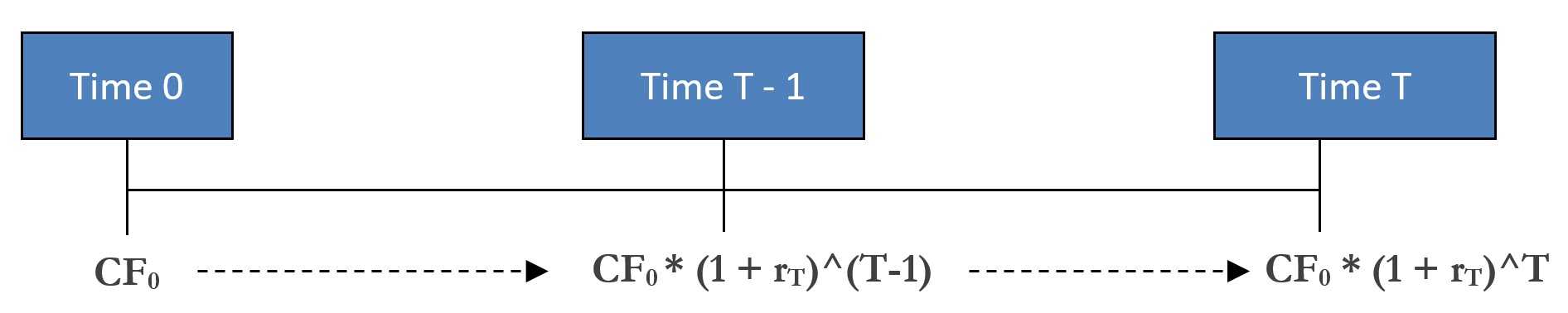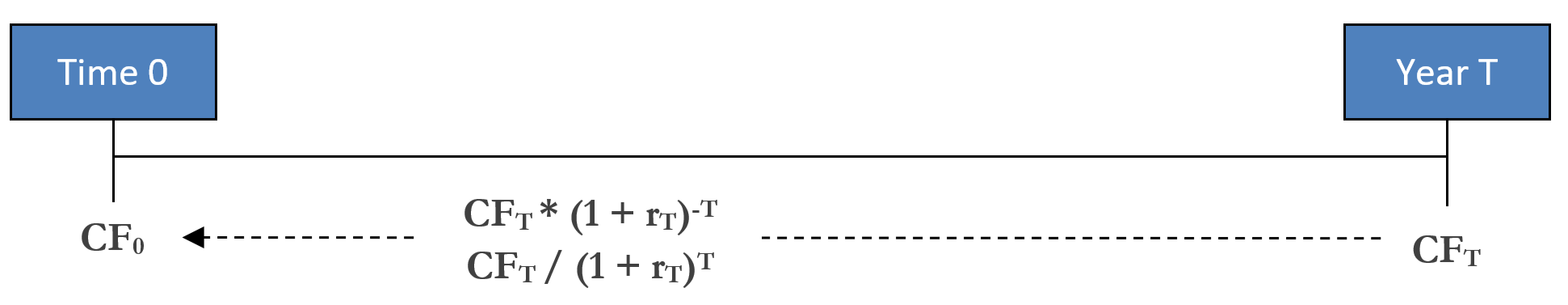# Prerequisite I: The Basics of Discounted Cash Flow & Net Present Value Analyses

Note: Below the Summary section are formatted, blank versions of the Excel Figures found in the full chapter of the hard copy textbook. There are exercises with instructions contained in the individual tabs of the file.

## Summary

Listen to this narration if you prefer

Discounted cash flow analysis (DCF) postulates that the value of a property is equal to its expected future cash flows discounted to present dollars. DCF is premised upon two basic concepts: the only source of value for a property is its ability to generate future cash flows; and, a dollar received today is more valuable than a dollar received tomorrow.  However, simply making these assumptions does not necessarily make them true.

While it is often true that future cash flows are the sole determinants of a property’s value, some investors value a property for more than its income potential.  For example, an investor can attribute a psychic value (as with a piece of art) to a property.  In other instances, pride of ownership will influence value (thus the expression “trophy property”).  If these reasons are reflected in future cash streams (i.e., the best building always sells for more), it fits the DCF methodology.  However, as is sometimes the case with trophy properties, if an investor values the “bragging rights” of ownership, they will pay more than the value indicated by DCF analysis.  Does this make them bad investors? Not if they value the “bragging rights.”

The second concept underpinning DCF is formally known as the time value of money, which states that a dollar amount received today (referred to as Time 0) is worth more than that same nominal dollar amount received tomorrow or at any other point in the future.  Why? Because if you have, for example, \$100 at Time 0 (the present value), you can invest those \$100 and expect to have more than \$100 in the future (the future value), as the investment is expected to yield a positive return..Generic equation for future value (FV) with a growth rate of r

Thus, other things equal, you would prefer a property to produce income earlier rather than later, and you “discount” dollars received in the future to reflect this preference.  The discount rate is the required expected annual return on an investment.  The discount rate is applied to expected future values to convert (reduce) them to their today’s dollars equivalent.Generic equation for present value (PV) with a discount rate of r

A common tool that is used to help make investment decisions is the net present value (NPV) metric.  The net present value of a project is equal to the present value of the cash flows the investment generates minus your initial investment.

## Spoken Glossary Available On Amazon Alexa

Already using Alexa? Just say “Alexa, Open Commercial Real Estate Glossary”

If not, download the free Alexa app below and then add the “Commercial Real Estate Glossary” skill by clicking here, and then say “Alexa, Open Commercial Real Estate Glossary”

To view the definition, click or press on the term. Repeat to hide the definition.

#### Time Value of Money

States that a dollar amount received today (referred to as Time 0) is worth more than that same nominal dollar amount received tomorrow or at any other point in the future.

#### Discounted Cash Flow Analysis (DCF)

Postulates that the value of a property is equal to its expected future cash flows discounted to present dollars; premised upon two basic concepts: 1) the only source of value for a property is its ability to generate future cash flows, 2) a dollar received today is more valuable than a dollar received tomorrow.

#### Present Value

Value in the present of a future projected amount of money, reduced by the appropriate discount rate.

#### Future Value

How much a dollar amount today is expected to be worth some time in the future.

A present value amount that appropriately takes into account the inherent risk in a cash flow stream.

#### Compound Return

The rate of return, usually expressed as a percentage, that represents the cumulative effect that a series of gains or losses have on an original amount of capital over a period of time.

#### Discount Rate

The required expected annual rate of return that is used to reduce future projected cash flows to their present values.  The discount rate for a property is theoretically composed of four factors: the long-term risk-free rate (approximated by the yield on a 10-year U.S. Treasury bond), expected economy-wide inflation, the risk premium associated with unexpected outcomes in the property’s NOI, and the risk premium associated with the property’s illiquidity relative to a 10-year Treasury bond.

#### Discount Factor

A factor which, when multiplied by a predicted future cash flow from a loan or some other form of debt, gives its present value.

#### Discounted

Future cash flows are valued at less than par value because of the time value of money.

#### Unlevered Cash Flows

Property-level cash flows irrespective of the financing structure.

#### Equity Value

The value of a property when all of its liabilities are subtracted.

#### Reversion / Terminal Value / Residual

Estimated property sale value; theoretically captures the value of all future cash flows beyond the point of sale; to estimate the sale value you will generally apply a cap rate to a stabilized NOI.